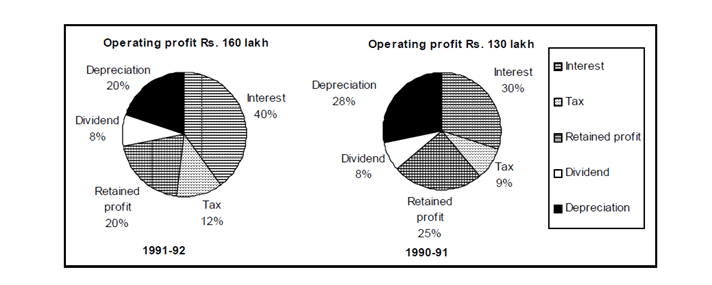Direction for questions 1 to 5: Answer the questions based on the following pie charts.Question 1: The operating profit in 1991-92 increased over that in 1990-91 by
a. 23%
b. 22%
c. 25%
d. 24%

Question 2: The interest burden in 1991-92 was higher than that in 1990-91 by
a. 50%
b. Rs. 25 lakh
c. 90%
d. Rs. 41 lakh

Question 3: If on an average, 5% rate of interest was charged on borrowed funds, then the total borrowed funds used by this company in the given two years amounted to
a. Rs. 221 lakh
b. Rs.1950 lakh
c. Rs. 568 lakh
d. Rs. 1030 lakh

Question 4: The retained profit in 1991-92, as compared to that in 1990-91 was
a. higher by 2.5%
b. higher by 1.5%
c. lower by 2.5%
d. lower by 1.5%

Question 5: The equity base of this company remained unchanged. Then the total dividend earning by the share holders in 1991-92 is
a. Rs. 124 lakh
b. Rs. 9 lakh
c. Rs. 10.4 lakh
d. Rs. 12.8 lakh

Operating profit in 1990-91 = 130 lakh

Operating profit in 1991-92 = 160 lakh

Percentage increase = (160 – 130)/130 × 100 = 300/13 = 23%

By analyzing the pie chart we can calculate that Interest in 1990-91 = 30% of 130 lakh = Rs. 39 lakh

Interest in 1991-92 = 40% of 160 lakh= Rs. 64 lakh

Hence, Interest burden in 1991-92 is greater than interest burden in 1990-91 by (64 – 39)lakh = Rs. 25 lakh

Total interest = sum of interest in 1990-91 and 1991-92 = (30% of 130) + (40% of 160) = (39 + 64)

= Rs. 103 lakh.

Now average rate of interest is 5% p.a. hence for two years the interest would be 5*2=10%

If borrowed fund is charged byinterest, then (10% of borrowed funds) = 103.

Hence, borrowed funds

= (10 × 103) = Rs. 1030 lakh.

From the given data in the pie chart

Retained profit in 1990-91 = (25% of 130) lakh= Rs. 32.5 lakh

Retained profit in 1991-92 = (20% of 160) lakh= Rs. 32 lakh

∴ Percentage change in retained profit = (32.5 – 32)/ 32.5 = 1.5% lower